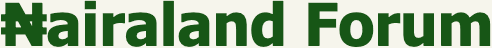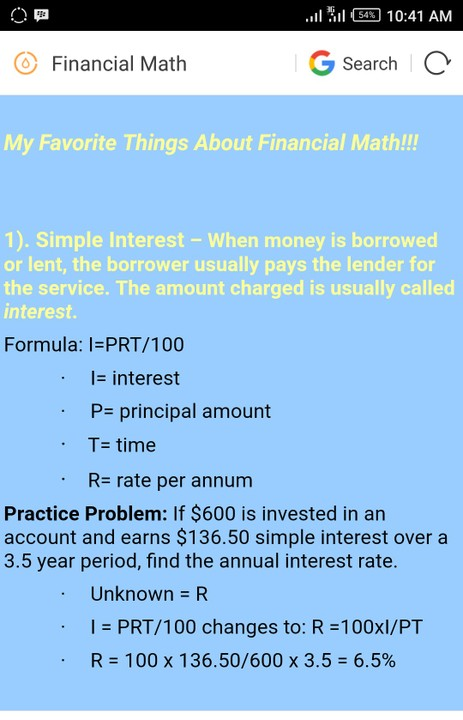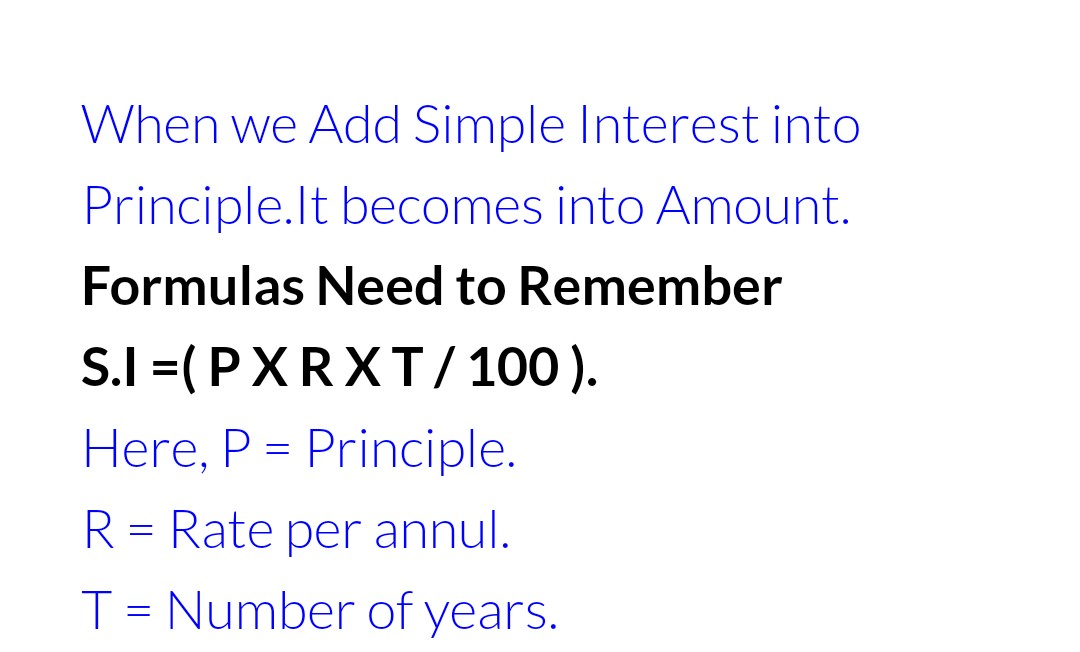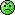#Welcome, Guest: Register On Nairaland / LOGIN! / Trending / Recent / New
Stats: 2,992,820 members, 7,290,761 topics. Date: Saturday, 04 February 2023 at 10:55 PM

## The Formula For Finding Simple Interest Is Not PRT/100. - Education - Nairaland

 The Formula For Finding Simple Interest Is Not PRT/100. by bhmw(m): 3:18pm On Jun 18, 2018 We were taught in basic and secondary school that the formula for finding simple interest is I = PRT/100. This is actually wrong as the formula for finding simple interest is I = PRT. Pass it across till it get to all stakeholders in the education sector so that this topic can be reviewed.Check out the video below for explanation. https://www.youtube.com/watch?v=5KobY0KuS4w Re: The Formula For Finding Simple Interest Is Not PRT/100. by Ocheinstein(m): 4:53pm On Jun 18, 2018 While I had no time and data to watch your video, I want you to know that the 100 there is simply put there as a part of R. Thus the rate is simply whatever is given expressed as a percentage.S.I =P *R%*TR% is simply R/100Weldone all the same 4 Likes Re: The Formula For Finding Simple Interest Is Not PRT/100. by bhmw(m): 6:27pm On Jun 18, 2018 If 100 is given as a constant in the formula and a rate is given as %(which means R/100) in a given question, then there will be 2 100's as denominators. Mathematically, what we have been solving as simple interest is simply wrong. Re: The Formula For Finding Simple Interest Is Not PRT/100. by bhmw(m): 10:52pm On Jun 18, 2018 Ocheinstein:While I had no time and data to watch your video, I want you to know that the 100 there is simply put there as a part of R. Thus the rate is simply whatever is given expressed as a percentage.S.I =P *R%*TR% is simply R/100Weldone all the sameMost countries no longer solve it as PRT/100 (which is incorrect), but PRT. Since mathematics is a universal subject, we need to also look into it and do the needful. Re: The Formula For Finding Simple Interest Is Not PRT/100. by femi4: 6:39am On Jun 19, 2018 bhmw:If 100 is given as a constant in the formula and a rate is given as %(which means R/100) in a given question, then there will be 2 100's as denominators. Mathematically, what we have been solving as simple interest is simply wrong. you are wrong. R is given as rate not rate in percentage. Hence, I = PRT/100If you define R as rate in percentage then I = PRTGet the grammar right! 1 Like Re: The Formula For Finding Simple Interest Is Not PRT/100. by sylvez(m): 7:56am On Jun 19, 2018 SI=PRT=ANSWERif you want to express the answer in percentage then you have : ANSWER/100 I don't really understand what op is trying to say, though I did not watch the video because my tecno spark is a bleeped up phone Re: The Formula For Finding Simple Interest Is Not PRT/100. by bhmw(m): 10:21am On Jun 19, 2018 femi4:you are wrong. R is given as rate not rate in percentage. Hence, I = PRT/100If you define R as rate in percentage then I = PRTGet the grammar right!You are the one who need to get the grammar right...Have you seen any question where the rate is give as ordinary figure without the symbol %?What is the meaning of that symbol %? For example 10% (it means 10/100 or 0.01).Check your simple interest questions then get back to me.You can also google out the formula for finding simple interest. Re: The Formula For Finding Simple Interest Is Not PRT/100. by bhmw(m): 10:30am On Jun 19, 2018 sylvez:SI=PRT=ANSWERif you want to express the answer in percentage then you have : ANSWER/100 I don't really understand what op is trying to say, though I did not watch the video because my tecno spark is a bleeped up phone In a given question, the rate is always given as % per annum, right? That symbol makes the rate a decimal or fraction. For example, find the simple interest on #5000 at 5% for 1 year.I = PRTP=#5000R= 5% (NOT 5) = 5/100 OR 0.05T= 1 YEARI = 5000 * 0.05 * 1 = #250If you divide it by another 100, the answer will be wrong. What I am saying in essence is that we should teach the topic the right way by interpreting the rate in a given question as fraction or decimal. Hope you understand now. Google it and get back to me. Re: The Formula For Finding Simple Interest Is Not PRT/100. by femi4: 10:33am On Jun 19, 2018 bhmw:You are the one who need to get the grammar right...Have you seen any question where the rate is give as ordinary figure with the symbol %?What is the meaning of that symbol %? For example 10% (it means 10/100 or 0.01).Check your simple interest questions then get back to me.You can also google out the formula for finding simple interest.Yes!Did I use "℅" in my other definition?Its the definition that you should followIf I tell you that I= PRT, I ll define R as rate in %But if I tell that I = PRT/100, then I ll define R as rate.Olodo!Example below:Re: The Formula For Finding Simple Interest Is Not PRT/100. by bhmw(m): 10:50am On Jun 19, 2018 femi4:Yes!Did I use "℅" in my other definition?Its the definition that you should followIf I tell you that I= PRT, I ll define R as rate in %But if I tell that I = PRT/100, then I ll define R as rate.Olodo!Must you insult me? No problem... In any given question, rate is always given in %, except you want to start forming your own question. Open your mind and learn. Once it is given in percent (%), it automatically mean a fraction or a decimal. Except if the question gives rate as an ordinary figure (and I am yet to see one). I said google it and get back. Don't insult me again or else I will not answer you again. Re: The Formula For Finding Simple Interest Is Not PRT/100. by bhmw(m): 11:01am On Jun 19, 2018 femi4:Yes!Did I use "℅" in my other definition?Its the definition that you should followIf I tell you that I= PRT, I ll define R as rate in %But if I tell that I = PRT/100, then I ll define R as rate.Olodo!Example below: In your given example, I=PRT/100, recall that you said if you use this formula, you R will be defined as rate (not in percent). The final answer therefore should not contain the symbol (%) (If this was done, then i will agree with you). Things are changing bro, the fact that we have been using it for long does not mean it cannot be changed. I can defend this to the latter. I have a video on how to calculate rate in a given question, I will post it soon. LEARN, UNLEARN AND RELEARN. Re: The Formula For Finding Simple Interest Is Not PRT/100. by alex81(m): 11:21am On Jun 19, 2018 All these is not necessary. It all comes down to how d terms(letters) in d formula is defined.Op instead forward ur energy in discovering/invention of new technology n new processes. Re: The Formula For Finding Simple Interest Is Not PRT/100. by bhmw(m): 11:24am On Jun 19, 2018 Where are the maths gurus in the house...Let us discuss this issue at hand. Re: The Formula For Finding Simple Interest Is Not PRT/100. by femi4: 11:31am On Jun 19, 2018 bhmw: In your given example, I=PRT/100, recall that you said if you use this formula, you R will be defined as rate (not in percent). The final answer therefore should not contain the symbol (%) (If this was done, then i will agree with you). Things are changing bro, the fact that we have been using it for long does not mean it cannot be changed. I can defend this to the latter. I have a video on how to calculate rate in a given question, I will post it soon. LEARN, UNLEARN AND RELEARN.Olodo! % means over 100. The final answer reads "R" = "Value in %" Re: The Formula For Finding Simple Interest Is Not PRT/100. by bhmw(m): 11:32am On Jun 19, 2018 alex81:All these is not necessary. It all comes down to how d terms(letters) in d formula is defined.Op instead forward ur energy in discovering/invention of new technology n new processes.I am teacher and I can point out things that are not done rightly in my profession. Mathematics is a universal subject. Simple interest formula cannot be two. We should apply what is universally accepted and teach it the right way (interpreting it to the latter). We should make our pupils/students understand the subject not just cramming.Anyway, in my field I have two board games I have created (awaiting for publication and distribution) Re: The Formula For Finding Simple Interest Is Not PRT/100. by bhmw(m): 11:39am On Jun 19, 2018 femi4:Olodo! % means over 100Thank you exactly what I am pointing out to you. You are contradicting yourself...maybe you should see the video.% means over 100 and should be interpreted as such in any given question (with explanation to the pupils/students). Check out my previous explanation maybe your OLODO square brain will pick something. Re: The Formula For Finding Simple Interest Is Not PRT/100. by bhmw(m): 11:45am On Jun 19, 2018 femi4:Olodo! % means over 100. The final answer reads "R" = "Value in %"I can see you are a learner... you said % means over 100 (i.e 6.5/100), can you work out the interest by substituting it into your formula I=PRT/100. Check out your answer with the value of interest in the question. Open your mind and see that mathematically, I=PRT and not I=PRT/100.Olodo square! Re: The Formula For Finding Simple Interest Is Not PRT/100. by alex81(m): 11:59am On Jun 19, 2018 bhmw:I am teacher and I can point out things that are not done rightly in my profession. Mathematics is a universal subject. Simple interest formula cannot be two. We should apply what is universally accepted and teach it the right way (interpreting it to the latter). We should make our pupils/students understand the subject not just cramming.Anyway, in my field I have two board games I have created (awaiting for publication and distribution) okay, while ur waiting for publication create an app for the game And forward a download link to me. leave this simple interest formula, u won't get an alert arguing over it here. Re: The Formula For Finding Simple Interest Is Not PRT/100. by femi4: 12:20pm On Jun 19, 2018 bhmw:I can see you are a learner... you said % means over 100 (i.e 6.5/100), can you work out the interest by substituting it into your formula I=PRT/100. Check out your answer with the value of interest in the question. Open your mind and see that mathematically, I=PRT and not I=PRT/100.Olodo square!Ode! I stated earlier that if I use R as x/100 or x%, my formula will be I = PRTBut if my R = x, then I = PRT/100Another example below, did you see ℅ in the definition of R?Re: The Formula For Finding Simple Interest Is Not PRT/100. by bhmw(m): 12:24pm On Jun 19, 2018 alex81: okay, while ur waiting for publication create an app for the game And forward a download link to me. leave this simple interest formula, u won't get an alert arguing over it here.Thanks... just want to bring it to the awareness of our publishers so that they can do the needful.Do you know who I can approach for the game to be turned to an app?Thanks once again. Re: The Formula For Finding Simple Interest Is Not PRT/100. by bhmw(m): 12:32pm On Jun 19, 2018 femi4:Ode! I stated earlier that if I use R as x/100 or x%, my formula will be I = PRTBut if my R = x, then I = PRT/100I won't give up on you, I will treat you as my student who is finding it real hard to readjust his brain...the fact that it was once like that does not mean that it cannot change (that is the issue you are having). You don't decide what you want to use as rate, the value of R is always "x%". I am yet to see where R is given as "x" (can you show me one).Since R is always given as x%, the formula remains I=PRT. You are a mathematician, get it right bro (calm down and stop insulting me) Re: The Formula For Finding Simple Interest Is Not PRT/100. by femi4: 12:34pm On Jun 19, 2018 bhmw:I won't give up on you, I will treat you as my student who is finding it real hard to readjust his brain...the fact that it was once like that does not mean that it cannot change (that is the issue you are having). You don't decide what you want to use as rate, the value of R is always "x%". I am yet to see where R is given as "x" (can you show me one).Since R is always given as x%, the formula remains I=PRT. You are a mathematician, get it right bro (calm down and stop insulting me)Answer the last question from the attachment. Re: The Formula For Finding Simple Interest Is Not PRT/100. by bhmw(m): 12:36pm On Jun 19, 2018 femi4:Answer the last question from the attachment. Can't see any question...type it. Re: The Formula For Finding Simple Interest Is Not PRT/100. by femi4: 12:48pm On Jun 19, 2018 bhmw:Can't see any question...type it.R = Rate per annum... You just suddenly gone blind. Did you see % in the definition.You are just impossible! I m done!Re: The Formula For Finding Simple Interest Is Not PRT/100. by bhmw(m): 12:50pm On Jun 19, 2018 femi4:Yes!Did I use "℅" in my other definition?Its the definition that you should followIf I tell you that I= PRT, I ll define R as rate in %But if I tell that I = PRT/100, then I ll define R as rate.Olodo!Example below:To answer this question, I=PRT, R=I/PTI=136.50P=600T=3.5R= X%=X/100By sustitution, X/100 = 136.50/600*3.5Cross multiply, you have 600*3.5*X = 136.50 *100X= 13650/600*3.5 = 6.5 (not 6.5 %)R= X% = 6.5%. Re: The Formula For Finding Simple Interest Is Not PRT/100. by bhmw(m): 12:57pm On Jun 19, 2018 femi4:R = Rate per annum... You just suddenly gone blind. Did you see % in the definition.You are just impossible! I m done!Must you insult me while expressing yourself? It shows the kind of person you are. In the principal, did you see the sign of money. It is just expression of the terms without the units. Re: The Formula For Finding Simple Interest Is Not PRT/100. by Nobody: 2:35pm On Jun 19, 2018★ ★ ★ ★ ★ ★ ★ ★ ★ ★∆ Una no ge work. aswear ∆★ ★ ★ ★ ★ ★ ★ ★ ★ ★ Re: The Formula For Finding Simple Interest Is Not PRT/100. by bhmw(m): 2:57pm On Jun 19, 2018 OrestesDante:★ ★ ★ ★ ★ ★ ★ ★ ★ ★∆ Una no ge work. aswear ∆★ ★ ★ ★ ★ ★ ★ ★ ★ ★Omo, I get work ooo... I be confirm teacher. I dey do am with passion. Things don change as per simple interest and he be like our publishers dem never notice. If na lie check for formula of simple interest. We need change this method... na wetin I dey try do be that ooo. No mind that my small friend. Re: The Formula For Finding Simple Interest Is Not PRT/100. by sylvez(m): 3:53pm On Jun 19, 2018 bhmw:In a given question, the rate is always given as % per annum, right? That symbol makes the rate a decimal or fraction. For example, find the simple interest on #5000 at 5% for 1 year.I = PRTP=#5000R= 5% (NOT 5) = 5/100 OR 0.05T= 1 YEARI = 5000 * 0.05 * 1 = #250If you divide it by another 100, the answer will be wrong. What I am saying in essence is that we should teach the topic the right way by interpreting the rate in a given question as fraction or decimal. Hope you understand now. Google it and get back to me.It's the same thing sir, SI=PRT/100therefore: 5000*5*1/100=N250 Re: The Formula For Finding Simple Interest Is Not PRT/100. by bhmw(m): 4:38pm On Jun 19, 2018 sylvez:It's the same thing sir, SI=PRT/100therefore: 5000*5*1/100=N250The rate is 0.05 not 5...that is the issue with this formula. We input rate as a whole number. This is wrong. Kindly research further. Re: The Formula For Finding Simple Interest Is Not PRT/100. by sylvez(m): 6:06pm On Jun 19, 2018 bhmw:The rate is 0.05 not 5...that is the issue with this formula. We input rate as a whole number. This is wrong. Kindly research further. so, your point is that rate is already expressed in percentage in a given question, so,it should be in decimal in solving.... no waha, I give up Re: The Formula For Finding Simple Interest Is Not PRT/100. by oxladexo(m): 8:34pm On Jun 19, 2018 bhmw:The rate is 0.05 not 5...that is the issue with this formula. We input rate as a whole number. This is wrong. Kindly research further. Mr teacher, you're confusing yourself.S.I is simply = PRT/100. If you say R should be in decimal, i.e., divided by 100 then the formula would be S.I=PRT.But in our schools R is taken as a whole number that's why it's PRT/100.If you take R as a decimal then still divide PRT by 100, then that's complete nonsense. So, it's the same.You choose to take R as a percentage and use PRTAnother person takes R as a whole number and uses PRT/100, you would both arrive at the same answer, and both solutions are correct. So it's no new discovery really, if that's the method you choose to teach your students, it's all good!